HomeBlogData Science5 Types of Binary Tree Explained [With Illustrations]

# 5 Types of Binary Tree Explained [With Illustrations]

Last updated:
28th Sep, 2022
Views
11 MinsView AllIn computer science, various data structures help in arranging data in different forms. Among them, trees are widely used abstract data structures that simulate a hierarchical tree structure. A tree usually has a root value and subtrees that are formed by the child nodes from its parent nodes. Trees are non-linear data structures.

A general tree data structure has no limitation on the number of child nodes it can hold. Yet, this is not the case with a binary tree. This article will learn about a specific tree data structure – binary tree and types of binary tree.

Check out our node js free course at upGrad

## What is Binary Tree Data Structure?

binary tree is a tree-type non-linear data structure with a maximum of two children for each parent. Every node in a binary tree has a left and right reference along with the data element. The node at the top of the hierarchy of a tree is called the root node. The nodes that hold other sub-nodes are the parent nodes.

A parent node has two child nodes: the left child and right child. Hashing, routing data for network traffic, data compression, preparing binary heaps, and binary search trees are some of the applications that use a binary tree.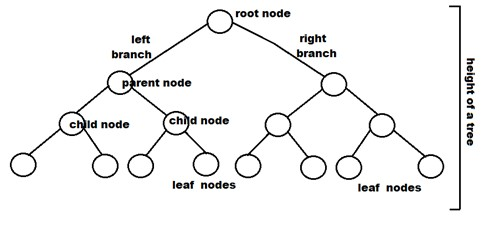## Terminologies associated with Binary Trees and Types of Binary Trees

• Node: It represents a termination point in a tree.
• Root: A tree’s topmost node.
• Parent: Each node (apart from the root) in a tree that has at least one sub-node of its own is called a parent node.
• Child: A node that straightway came from a parent node when moving away from the root is the child node.
• Leaf Node: These are external nodes. They are the nodes that have no child.
• Internal Node: As the name suggests, these are inner nodes with at least one child.
• Depth of a Tree: The number of edges from the tree’s node to the root is.
• Height of a Tree: It is the number of edges from the node to the deepest leaf. The tree height is also considered the root height.

Our learners also read: Excel online course free!

As you are now familiar with the terminologies associated with the binary tree and types of binary tree, it is time to understand the binary tree components. Check out our data science courses to learn in-depth about binary structure and components.

Our learners also read: Data structures and Algorithms free!

## Understanding Properties of Binary Tree Or What Is Binary Tree?

At every level of it, the maximum number allowed for nodes stands at 2i.

The height of a binary tree stands defined as the longest path emanating from a root node to the tree’s leaf node.

What Is Binary Tree– More Than The Binary Tree Definition

Say a binary tree placed at a height equal to 3. In that case, the highest number of nodes for this height 3 stands equal to 15, that is, (1+2+4+8) = 15. In basic terms, the maximum node number  possible for this height h is (20 + 21 + 22+….2h) = 2h+1 -1.

Now, for the minimum node number that is possible at this height h, it comes as equal to h+1.

If there are a minimum number of nodes, then the height of a binary tree would stand aa maximum. On the other hand, when there is a number of a node at its maximum, then the binary tree m height will be minimum. If there exists around ‘n’ number nodes in a binary tree, here is a calculation to clarify the binary tree definition.

The tree’s minimum height is computed as:

n = 2h+1 -1

n+1 = 2h+1

Taking log for both sides now,

log2(n+1) = log2(2h+1)

log2(n+1) = h+1

h = log2(n+1) – 1

The highest height will be computed as:

n = h+1

h= n-1

## Binary Tree Components

There are three binary tree components. Every binary tree node has these three components associated with it. It becomes an essential concept for programmers to understand these three binary tree components:

1. Data element
2. Pointer to left subtree
3. Pointer to right subtree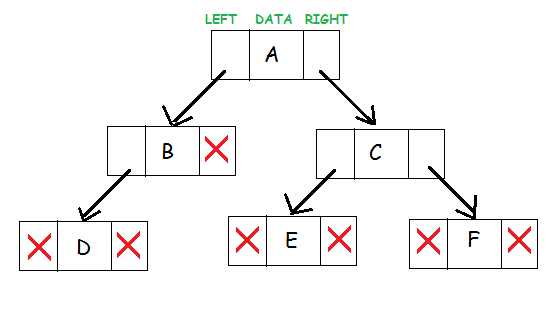Source

These three binary tree components represent a node. The data resides in the middle. The left pointer points to the child node, forming the left sub-tree. The right pointer points to the child node at its right, creating the right subtree.

## Explore our Popular Data Science Courses

 Executive Post Graduate Programme in Data Science from IIITB Professional Certificate Program in Data Science for Business Decision Making Master of Science in Data Science from University of Arizona Advanced Certificate Programme in Data Science from IIITB Professional Certificate Program in Data Science and Business Analytics from University of Maryland Data Science Courses

## Binary Tree Definition: An in-depth analysis

In case there exists n number of nodes in any binary tree, the height stands given by log n logn. This is due to the simple reason that for any given node in any binary tree, there will be two child nodes at the most. This drives us to an explanation to define binary tree: for every level or every height of any binary tree, the node number must be the same as around half the node numbers present at the next level.

In the form of an answer to define binary tree, the node number at every level is close to double the node number at its previous level. We hope this clears the answer to what is binary tree!

It means, that when a binary tree comes with height h, the number of nodes for define binary tree is  n = (2 ^ 0) + (2 ^ 1) + (2 ^ 2) + (2 ^ 3) + ….. + (2 ^ (h-1))(20)+(21)+(22)+(23)+…..+(2(h−1))

From the `mathematical induction point for what is a binary tree, this is what we know-

(2 ^ 0) + (2 ^ 1) + (2 ^ 2) + (2 ^ 3) + ….. + (2 ^ {(h-1)}) = (2 ^ h)-1(20)+(21)+(22)+(23)+…..+(2(h−1))=(2h)−1

Hence,

(2 ^ h)-1 = n => 2 ^ h = n + 1 => h = log2(n+1)(2h)−1=n=>2h=n+1=>h=log2(n+1)

Therefore, the minimum height for a binary tree is roughly equal to log(n) roughly. This helps you better understand what is a binary tree.

Also, the minimum number of nodes that are possible at height h of the binary tree can be known by h+1.

If the binary tree comes with an L number for leaf nodes, the height is represented by L + 1.

## Types of Binary Trees

There are various types of binary trees, and each of these binary tree types has unique characteristics. Here are each of the binary tree types in detail:

### 1. Full Binary Tree

It is a special kind of a binary tree that has either zero children or two children. It means that all the nodes in that binary tree should either have two child nodes of its parent node or the parent node is itself the leaf node or the external node.

In other words, a full binary tree is a unique binary tree where every node except the external node has two children. When it holds a single child, such a binary tree will not be a full binary tree. Here, the quantity of leaf nodes is equal to the number of internal nodes plus one. The equation is like L=I+1, where L is the number of leaf nodes, and I is the number of internal nodes.

Our learners also read: Python free courses!

Here is the structure of a full binary tree: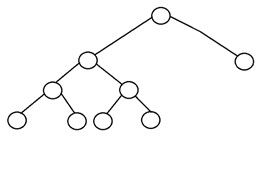## Top Data Science Skills to Learn

 Top Data Science Skills to Learn 1 Data Analysis Course Inferential Statistics Courses 2 Hypothesis Testing Programs Logistic Regression Courses 3 Linear Regression Courses Linear Algebra for Analysis

### 2. Complete Binary Tree

A complete binary tree is another specific type of binary tree where all the tree levels are filled entirely with nodes, except the lowest level of the tree. Also, in the last or the lowest level of this binary tree, every node should possibly reside on the left side. Here is the structure of a complete binary tree: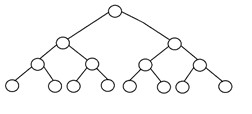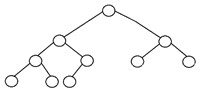upGrad’s Exclusive Data Science Webinar for you –

Transformation & Opportunities in Analytics & Insights

### 3. Perfect Binary Tree

A binary tree is said to be ‘perfect’ if all the internal nodes have strictly two children, and every external or leaf node is at the same level or same depth within a tree. A perfect binary tree having height ‘h’ has 2h – 1 node. Here is the structure of a perfect binary tree: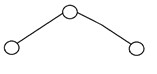### 4. Balanced Binary Tree

A binary tree is said to be ‘balanced’ if the tree height is O(logN), where ‘N’ is the number of nodes. In a balanced binary tree, the height of the left and the right subtrees of each node should vary by at most one. An AVL Tree and a Red-Black Tree are some common examples of data structure that can generate a balanced binary search tree. Here is an example of a balanced binary tree: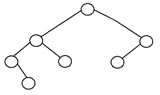### 5. Degenerate Binary Tree

A binary tree is said to be a degenerate binary tree or pathological binary tree if every internal node has only a single child. Such trees are similar to a linked list performance-wise. Here is an example of a degenerate binary tree: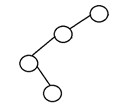## Benefits of a Binary Tree

• The search operation in a binary tree is faster as compared to other trees
• Only two traversals are enough to provide the elements in sorted order
• It is easy to pick up the maximum and minimum elements
• Graph traversal also uses binary trees
• Converting different postfix and prefix expressions are possible using binary trees

## Conclusion

The binary tree is one of the most widely used trees in the data structure. Each of the binary tree types has its unique features. These data structures have specific requirements in applied computer science. We hope this article about types of binary trees was helpful. upGrad offers various courses in data science, machine learning, big data, and more.

## Read our popular Data Science Articles

 Data Science Career Path: A Comprehensive Career Guide Data Science Career Growth: The Future of Work is here Why is Data Science Important? 8 Ways Data Science Brings Value to the Business Relevance of Data Science for Managers The Ultimate Data Science Cheat Sheet Every Data Scientists Should Have Top 6 Reasons Why You Should Become a Data Scientist A Day in the Life of Data Scientist: What do they do? Myth Busted: Data Science doesn’t need Coding Business Intelligence vs Data Science: What are the differences?

If you are curious to learn about data science, check out IIIT-B & upGrad’s Executive PG Program in Data Science which is created for working professionals and offers 10+ case studies & projects, practical hands-on workshops, mentorship with industry experts, 1-on-1 with industry mentors, 400+ hours of learning and job assistance with top firms.#### Rohit Sharma

Blog Author
Rohit Sharma is the Program Director for the UpGrad-IIIT Bangalore, PG Diploma Data Analytics Program.
Get Free Consultation

Select CourseSelectBy clicking 'Submit' you Agree to

#### Data Science Skills to Master

1What are the drawbacks of using a binary search tree?

It uses a recursive method that takes up more stack space. The binary search method is error-prone and complex to programme. Binary search has a bad relationship with memory hierarchy, i.e. caching.

2What is the use of a height-balanced binary tree?

Performing operations on balanced binary trees is computationally efficient. The following are the criteria for a balanced binary tree: At every given node, the absolute difference between the heights of the left and right subtrees is less than one. A balanced binary tree represents the left subtree of each node. Dealing with random values is frequently impossible in the real world, and the likelihood of dealing with non-random values (such as sequential) leads to skew trees, which is the worst case scenario. As a result, rotations are used to achieve height equilibrium.

3What is a binary tree's maximum height?

A binary tree's height is equal to the height of the root node in the whole binary tree. It means that the maximum number of edges from the root to the farthest leaf node determines the height of a binary tree. In a binary search tree, a node's left child has a lower value than the parent, while the right child has a higher value. When there are n nodes in a binary search tree, the greatest height is n-1 and the least height is floor (log2n).

## Suggested Blogs

295351
Summary: In this Article, you will learn about 4 Types of Data Qualitative Data Type Nominal Ordinal Quantitative Data Type Discrete Continuous R